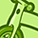Announcements
MAINTENANCE ALERT: Dec. 12th starting 9 AM CET. Community will be read-only. GET DETAILS
cancel
Showing results for
Did you mean:Not applicable

## group modifiers in aggregated expression

Hi all,

I have a problem. I tried to use these expressions in a chart:

sum({\$<[Id_Filiale]=,[id_categoria]=,[Nome_Dipendente_Cons]=>} aggr(count(Mese), Matricola_Dipendente, Anno_C)/12)

or

sum({\$<[Id_Filiale]=,[id_categoria]=,[Nome_Dipendente_Cons]=>} \$(vVal))

.... where vVal is a variable defined as: aggr(count(Mese), Matricola_Dipendente, Anno_C)/12

but they don't work! Someone can help me?
Thanks!

1 Solution

Accepted SolutionsNot applicable
Author

Hi Marcodesa,

actually I misunderstood the post of somenathroy, but he is actually right, the formula does work if you use the filter *both* in count and in sum as that:

sum({\$<[Id_Filiale]=,[id_categoria]=,[Nome_Dipendente_Cons]=>} aggr(count({\$<[Id_Filiale]=,[id_categoria]=,[Nome_Dipendente_Cons]=>}Mese), Matricola_Dipendente, Anno_C)/12)

I tried with my formula and it works!

let me know if it works for you.

bye,

azzurra

7 RepliesCreator III

hi,

the problem is with the "/12" part

the result of the aggr() function is not a single value but a series of values that are then passed to the enclosing sum() functions

and your trying to divide it by 12 which cant work

i dont know what exactly your trying to achieve but try moving the "/12" somewhere else

maybe like this

sum({\$<[Id_Filiale]=,[id_categoria]=,[Nome_Dipendente_Cons]=>} aggr(count(Mese/12), Matricola_Dipendente, Anno_C))

or like this

sum({\$<[Id_Filiale]=,[id_categoria]=,[Nome_Dipendente_Cons]=>} aggr(count(Mese), Matricola_Dipendente, Anno_C))/12Not applicable
Author

The problem is that this instruction doesn't work {\$<[Id_Filiale]=,[id_categoria]=,[Nome_Dipendente_Cons]=>}. For example if I select a value of the field "Id_Filiale", this expression aggr(count(Mese), Matricola_Dipendente, Anno_C)/12)  is filtered for that valueCreator III

so include {\$<[Id_Filiale]=,[id_categoria]=,[Nome_Dipendente_Cons]=>} also into Count( ) function.

Regards,

SomNot applicable
Author

no workNot applicable
Author

I have the same problem, trying to use aggregate functions with group modifiers, they seem not working with an expression or variable, like the one marcodesa has posted here.

So, as he said, if you select 'Id_Filiale' the expression is filtered by that value, but it should not!

While if you have an expression like this:

sum({\$<[Id_Filiale]=,[id_categoria]=,[Nome_Dipendente_Cons]=>} Sales)

it works without any problem.

Someone has any idea?Not applicable
Author

Hi Marcodesa,

actually I misunderstood the post of somenathroy, but he is actually right, the formula does work if you use the filter *both* in count and in sum as that:

sum({\$<[Id_Filiale]=,[id_categoria]=,[Nome_Dipendente_Cons]=>} aggr(count({\$<[Id_Filiale]=,[id_categoria]=,[Nome_Dipendente_Cons]=>}Mese), Matricola_Dipendente, Anno_C)/12)

I tried with my formula and it works!

let me know if it works for you.

bye,

azzurraNot applicable
Author

Hi Marcodesa,